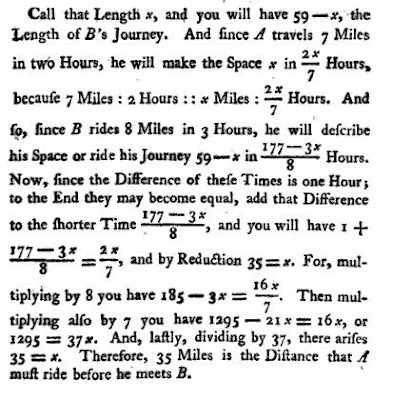## March 11, 2008

### Newton's Algebra Book

PROBLEM V. If two Post-Boys A and B, at 59 Miles Distance from one another, set out in the Morning to meet. And A rides 7 Miles in two Hours, and B 8 Miles in three Hours, and B begins his Journey an Hour later than A; to find what Number of Miles A will ride before he meets B.Math teachers would probably find the substance of this word problem, if not its expression, to be comfortably familiar. They may be surprised to learn, however, that this particular problem comes from the 1769 edition of an English translation of a textbook written in Latin by Isaac Newton (1643–1727).

Celebrated as the inventor of calculus and the author of Philosophiae Naturalis Principia Mathematica (Mathematical Principles of Natural Philosophy), Newton also prepared lecture notes during the time that he was at the University of Cambridge as the Lucasian Professor of Mathematics. All undergraduates were required to attend the Lucasian lectures, starting in their third year.

In 1669, Newton had made extensive notes on an algebra textbook written by Gerard Kinkhuysen and published in 1661. Like other 17th-century textbooks on solving equations, Kinkhuysen's book built on traditional material from the previous century, with the addition of some new ideas from René Descartes and Frans van Schooten.

Newton used these notes later when he compiled his Lucasian lectures, organizing the material in much the same way that Kinkhuysen had in his book. It seems unlikely that Newton ever delivered the lectures, but William Whiston (1667–1752), Newton's successor as Lucasian Professor, edited and published the notes in 1707 as the book Arithmetica universalis.

Newton himself was unhappy with the publication of his notes and refused to let his name appear on the book. When the book was printed, he apparently threatened to buy all the copies so that he could destroy them. The book was later translated into English by Joseph Raphson (1648–1715) and published in 1720 as Universal arithmetick.

A second, amended edition of Arithmetica universalis was published in 1722. Newton's name first appears on the 1761 edition of Arithmetica universalis, printed well after his death.

Arithmetica universalis covers a wide range of elementary topics, including algebraic notation, arithmetic, the relationship between geometry and algebra, limits and infinite series, the binomial theorem, and the solution of equations, including the extraction of roots.

Because Newton's book closely follows the structure and content of Kinkhuysen's Algebra, it has "the appearance of being far more elementary than in fact it is," writes Jacqueline A. Stedall of The Queen's College, Oxford. "To the traditional ideas expounded by Kinkhuysen, Newton added some far-reaching insights of his own."

Nonetheless, she adds, Newton often "described rules or procedures without explanation, giving rise to extensive commentary later on."

Today's high school teachers could profit from pondering the way Newton handled fundamental operations and questions of arithmetic and the wide array of examples and problems that he posed, says mathematician Michel Helfgott of East Tennessee State University.

Helfgott and George Baloglou of the State University of New York at Oswego themselves went back to Newton's Universal arithmetick when they recently investigated relations among a triangle's area, perimeter, and angles. They generalized Newton's derivation of the formula x = P/2 – 2A/P, expressing a right triangle's hypotenuse, x, in terms of its area, A, and perimeter, P. The results are in their paper "Angles, Area, and Perimeter Caught in a Cubic," published earlier this year in Forum Geometricorum.

Here's Newton's solution to the problem posed at the beginning of this article, as published in the 1769 edition of Universal arithmetick.